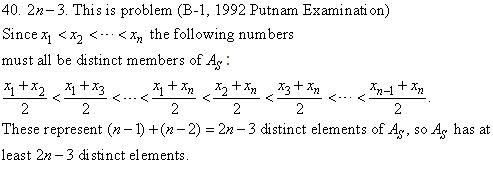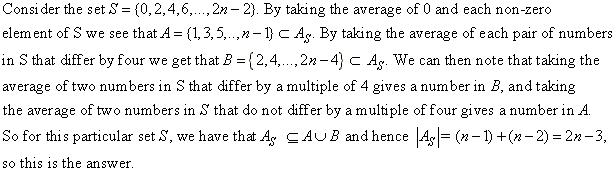20th Annual Lehigh University / AT&T High School Math Contest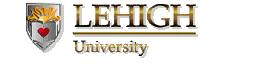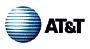March 4, 2000

Solutions
Please note the corrections in red.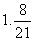2. 0

3. 7 . Find the point of intersection of the lines 5r+7b = 69 and 9r+3b=57, which is (r,b) = (4,7)

4. 3/4.A line L, between the orgin and the point (3,4) is perpendicular to the line we
want to find. Since L has slope 4/3, the slope of the given line is 3/4.

5. 7. The sum of any two sides of a triangle must be larger than the third side. The smallest value satisfying this constraint is 7.

6. 69 cents.

7.  y = -2x + 6

8.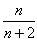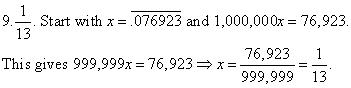10.  21. We will have one of two cases.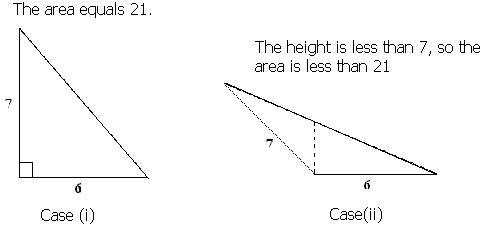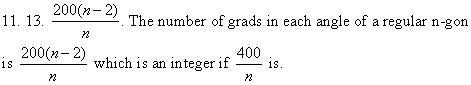12. 22000 - 1. Every game has exactly 1 loser, so at any point during the tournament the number of
games played equal the number of losers.  There are 22000 players in the tournament and only one
winner. Hence there are 22000 - 1 losers.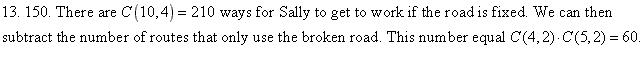14. 3.  Divide the chips into three groups: A: the coins on the left platform, B: the chips on the
right platform and C: the coins on neither platform. Start with A=5, B=5 and C=6.
If weight(A) < weight(B) then the fake coin is in set A, if weight(A) > weight(B) then the
fake coin is in set B. If weight (A) = weight(B) then the fake coin is in set C. After the
first weighing we are able to eliminate at least 10 coins from consideration. We then take
A = 2, B=2, and the rest in C. If weight (A)<weight(B) then the fake coin is in A, if
weight (A) > weight(B) the fake coin is in B, and if weight(A) = weight(B) then the fake
coin is in set C. After the second weighing we can eliminate all but two coins, and these
can be placed on scale to determine the fake one.

15. 10. The number of seconds in 6 weeks equals 6 * 7 * 24 * 60 * 60 =
6 * 7 * 24 * 30 * 5! = 24 * 30 * 7 = 10!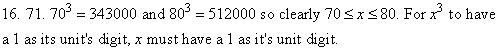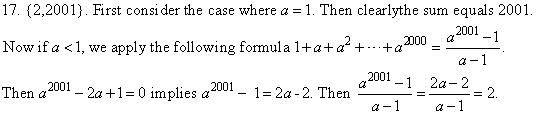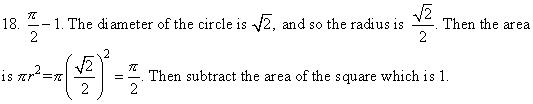19. 5. Going from step 4 to 5 is not valid since it requires dividing by (a-b)=0.

Note the answer is  -1000/3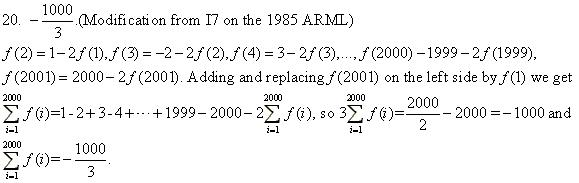21. 12,960 = 3*3!*6!   We must have one of the following three cases where the
T's represent teachers and the S's represent students:
(i) T S S T S S T S S  (ii) S T S S T S S T S (iii) S S T S S T S S T
In each of these cases there are 3! ways to arrange the teachers and 6! ways to
arrange the students. The answer is then 3*3!*6!.

22. 5040. 10*9*8*7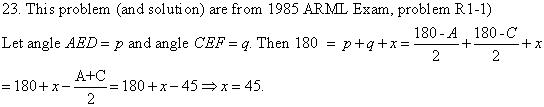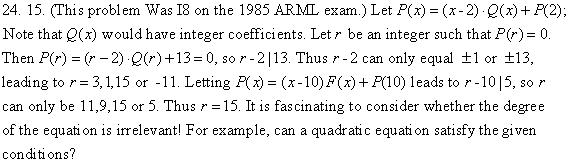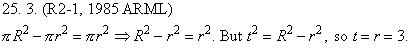Note this is t = r = 3, not t = r = 2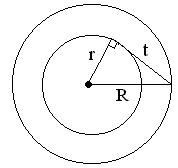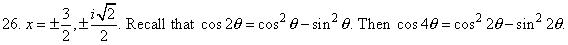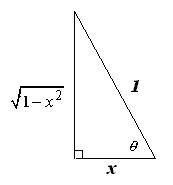The diagram above gives us that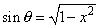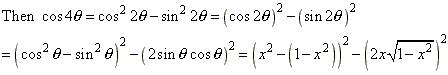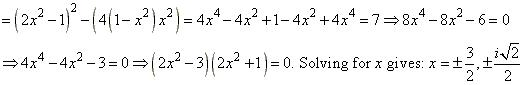27. (2,2000). (Modification from R1-5, 1985 ARML) Each line is of the form
y = mx + b = mx + (2000 - 2m), or y  t = m (x-2). This is the "point-slope" form of a line,
and all these lines go through (2,2000). It is interesting to consider sets of lines whose slope
and y - intercept are related in a given ways. For example what property has the set of lines
for which m2 = b?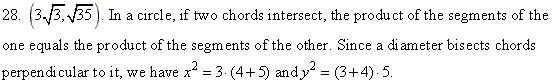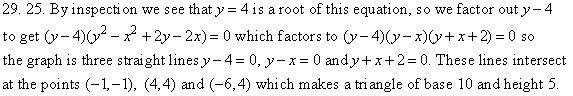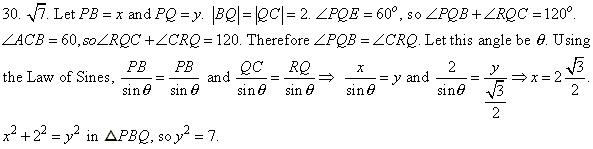31. 1,499,500. Suppose 24y+1= x224y + 1 is not divisible by 2 or 3, so x is not divisible by 2 or 3. 24y - x2  1 = (x + 1)( x - 1). If x is not divisible by 2, then one of x+1 and x-1 is divisible by 2 and the other is divisible by 4. If x is not divisible by 3, then exactly one of x+1 or x-1 is. Then x2-1 is divisible by 24, so there is one y for every x not divisible by 2 or 3. the 2000th such x is 5999. So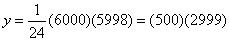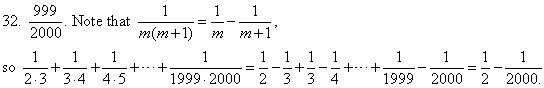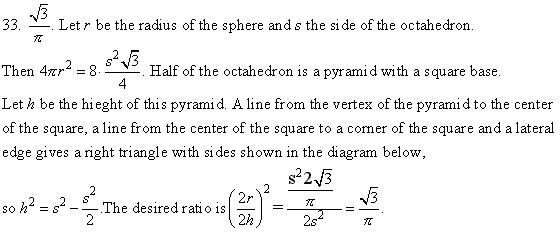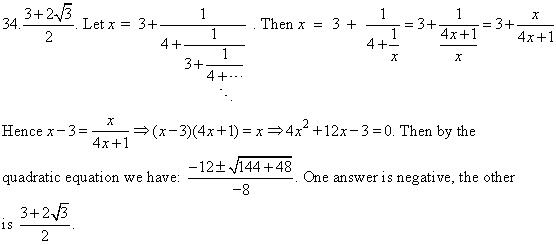35.  3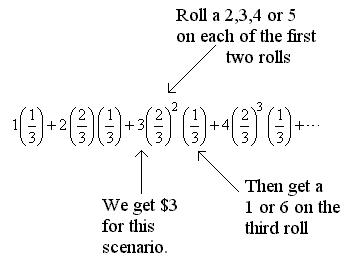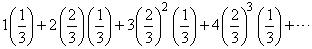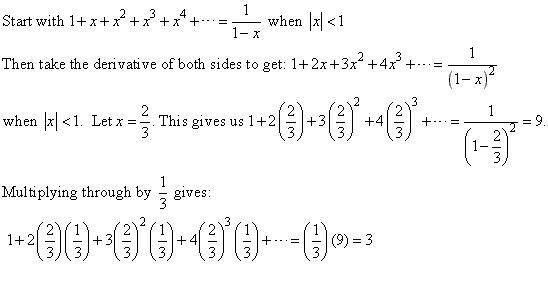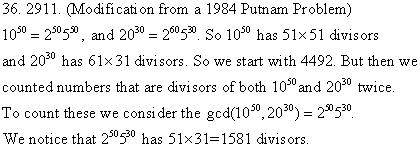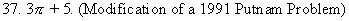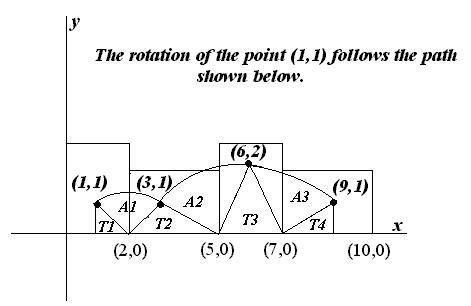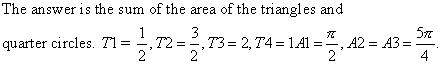38.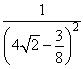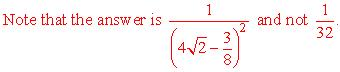To win the center of the penny must lie in the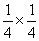square as shown in the circle on the right.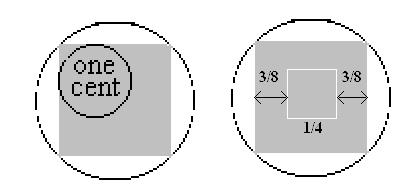So the winning space is 16 * (1/16) = 1 square units, and the center of the penny can
be anywhere on or inside the frame.

If the entire penny must lie on the board which has dimensions: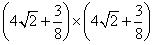the center of the penny must lie in a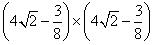square.

39. 233. Let An be the number of different tilings of an 1 x n rectangle. So A1 = 1 and
A2 = 2. Any tiling of An where n > 2 must begin with a 1x1 gray square or a 1x2 black
rectangle. Hence we have that An =  An-1+An-2   This gives us the Fibonacci numbers
A1 = 1, A2 = 2, A3 = 3, A4= 5,, A12 = 233.